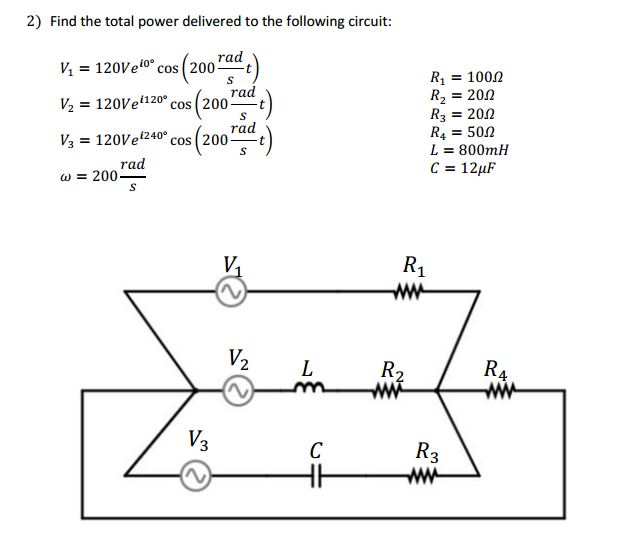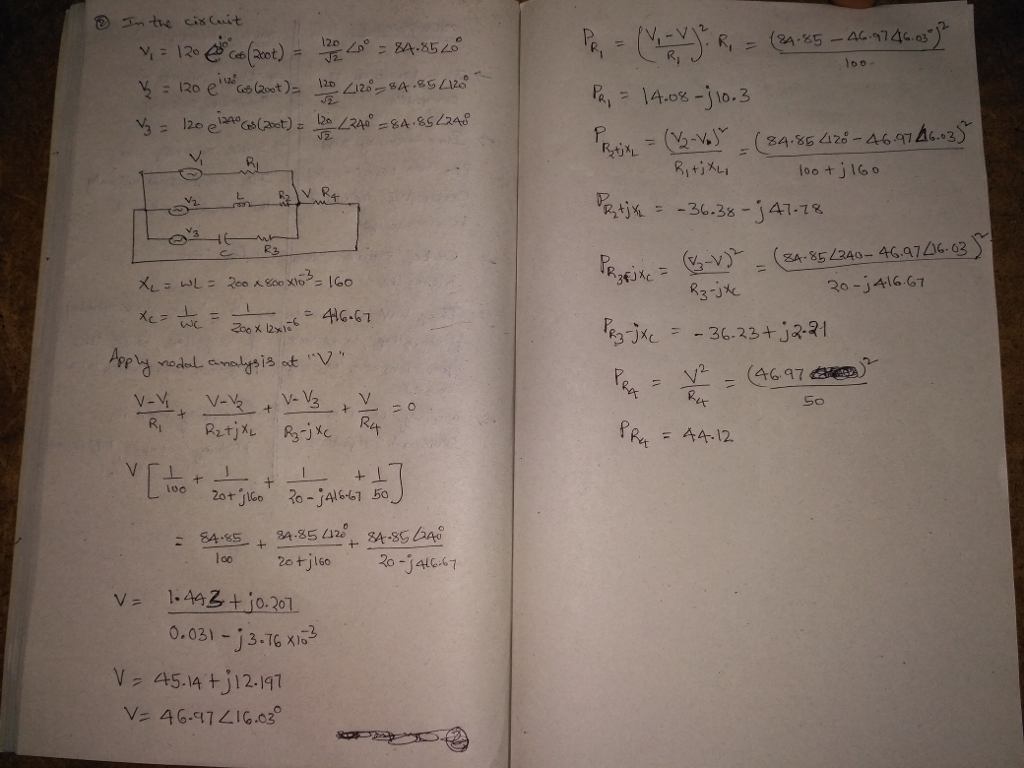# Homework Solution: Find the total power delivered to the following circuit: V_1 = 120V e^i 0 degree cos(200 rad/s t) V_2 = 120V e^i 120 degree cos (…Find the total power delivered to the following circuit: V_1 = 120V e^i 0 degree cos(200 rad/s t) V_2 = 120V e^i 120 degree cos (200 rad/s t) V_3 = 120V e^i 240 degree cos (200 rad/s t) omega = 200 rad/s R_1 = 100 ohm R_2 = 20 ohm R_3 = 20 ohm R_4 = 50 ohm L = 800 mu H C = 12 mu F